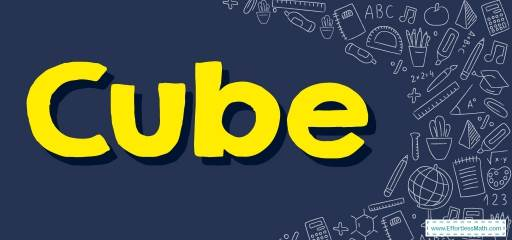# How to Find Volume and Surface Area of Cubes? (+FREE Worksheet!)

Here you can learn how to find the volume and surface area of cubes using cubes volume and surface area formulas.## Step-by-step guide to finding the Volume and Surface Area of Cubes

• A cube is a three-dimensional solid object bounded by six square sides.
• Volume is the measure of the amount of space inside of a solid figure, like a cube, ball, cylinder, or pyramid.
• Volume of a cube $$=$$ (one side)$$^3$$
• surface area of cube $$=6×$$ (one side)$$^2$$

The Absolute Best Book for 4th Grade Students

### Cubes – Example 1:

Find the volume and surface area of this cube.Solution:

Use volume formula: volume $$=$$ (one side)$$^3$$
Then: volume $$=$$ (one side)$$^3=(4)^3=64$$ $$cm^3$$
Use surface area formula: surface area of cube: $$=6$$ (one side)$$^2$$
Then: surface area of cube $$=6$$ (one side)$$^2=6(4)^2=6(16)=96$$ $$cm^2$$

### Cubes – Example 2:

Find the volume and surface area of this cube.Solution:

Use volume formula: volume $$=$$ (one side)$$^3$$
Then: volume $$=$$ (one side)$$^3=(2)^3=8$$ $$cm^3$$
Use surface area formula: surface area of cube: $$=6$$ (one side)$$^2$$
Then: surface area of cube $$=6$$ (one side)$$^2=6(2)^2=6(4)=24$$ $$cm^2$$

## Exercises for Finding Volume and Surface Area of Cubes

The Absolute Best Book to Ace Grade 8 Math

### Find the volume of each cube.

1.2.3.4.1. $$\color{blue}{8 \ ft^3}$$
2. $$\color{blue}{125 \ m^3}$$
3. $$\color{blue}{27 \ in^3}$$
4. $$\color{blue}{216 \ miles^3}$$

The Best Math Books for Elementary Students

### What people say about "How to Find Volume and Surface Area of Cubes? (+FREE Worksheet!) - Effortless Math: We Help Students Learn to LOVE Mathematics"?

No one replied yet.

X
51% OFF

Limited time only!

Save Over 51%

SAVE $15 It was$29.99 now it is \$14.99